# Numb3rs 216: Protest

The only significant mathematical content in this episode was a brief discussion of social networking. We'll talk about an example of an experiment involving social networking and of a mathematical method to identifying important nodes in social networks.

## Social Networks

The basic idea of social networks is to represent the interactions beween a group of people by a mathematical structure called a graph. A graph is given by a set of vertices (also called nodes), and a set of pairs of vertices, called edges. To model friendships (or other types of interactions) among a group of people, we have one node for each person and an edge between each pair of people who are friends. Now we can talk about various quantities related to the graph or particular vertices. For example, we can count the number of edges at each vertex, the distance between two different vertices, etc. Here is an example graph.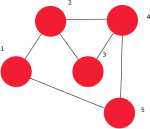Another way we can represent a graph is using matrices. If you aren't familiar with matrices, they came up in another Numb3rs show and are described here. If there are n vertices in the graph, we can number them 1 through n and then make a matrix where the number in row r and column c is 1 if there is an edge between vertex r and c and 0 otherwise. Notice that this matrix will be symmetric, which means that if you flip the matrix across the diagonal from the upper left to the lower right, you get the same matrix. The matrix representation for a graph can be useful for some types of computations. The matrix below represents the graph illustrated above.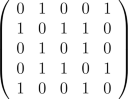Describing social networks mathematically has allowed various types of math to be applied. This has been done for many various applications, including uncloaking criminal networks amongst slumlords, revealing how hospital-acquired infection[HAI] spread amongst patients and medical staff, finding emerging leaders in fast growing companies, studying the spread of HIV in a prison system, expose business ties and financial flows to investigate possible criminal behavior, etc. As another example, researchers interviewed 800 students in a high school in the Midwest and asked them who they had had sexual experiences with. Then they drew a graph where the nodes were people and people who had had sexual experiences with each other were connected with an edge.

Activity 1:
1. What conclusions can you draw from the graph of the sexual relations at the high school? How should this graph affect how students are educated about STDs?
2. What other ways do you think social network analysis could be applied?

## Stanley Milgram Experiment

In the early 1960s Stanley Milgram was interested in testing the "Six Degrees of Seperation" hypothesis, which states that if you look at the social network of the whole world, then there is a path between any two nodes that has length at most 6.

The hypothesis that Milgram was testing is actually known to be false because there are some societies that actively avoid contact with the rest of the world. An example of this is the Sentinelese, a tribe of a few dozen to a few hundred people on a small island near India. They have been very hostile to any visitors, so very little is known about them at all. In particular, as far as anybody knows none of the people on the island are friends with anyone off the island.
He decided to test this hypothesis by mailing out letters to many random people. The letters told the recipients that they were supposed to try to mail the letter to somebody in Massachussetts, but that they were only allowed to mail it to someone they knew on a first name basis. If they didn't know the final person, they were supposed to mail it to someone they knew who would be more likely to know the person. At each step in the process, they were supposed to mail a postcard to Milgram so that he could record the steps that were taken. One obvious problem is that many people didn't send the letters on. In one of his experiments, 160 letters were mailed out, but only 24 reached the target. Among the ones that reached the target, the average path length was about 6.

Activity 2:
If you assume that at each node there's a certain probability p that the node won't send on the letter, then short paths are more likely to be completed than long paths.
1. If a path has length n (i.e. it crosses n edges), what is the probability that it is completed?
2. Let's say that given a starting point in the graph, the odds that the path from the point to the final point has length n are given by the formula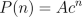, where A,c are constants and 0 < c < 1. (We choose A so that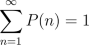.) What is the average length of a path in the network?
3. Using the formulas in the previous question, what is the average length of a completed path?

Another problem with Milgram's study is that people had to choose which one of their friends to send the letter to. Since they couldn't possibly know all of their friends connections, they weren't able to choose the most efficient route. In fact, from looking at the data it seems that most people decided to send the letter to one of their friends that was geographically closer to the target then they were. Once the letter arrived in the right town, it often moved around fairly randomly until it happened to get to one of the target's friends. This would cause Milgram's experiment to overestimate the minimum length of a path between two random people. Even with these difficulties, this experiment has encouraged much research into networks and graphs, particularly graphs with the small world property. This is the property that any two nodes in the graph can be connected by a short path. In a paper in 1998, Duncan Watts and Steven Strogratz wrote a paper that many networks, both natural and manmade, are small world networks. They also showed that if you start with a lattice and add a few random edges that the diameter (length of the longest path) of the graph goes from being very long to very short. This is a common occurance in mathematics - often there is experimental evidence to support some conjecture, but an actual proof of it can take a very long time to be discovered.

## Eigenvector Centrality

In the last section we talked about one measurement of graphs, and social networks in particular, the average distance between nodes. In this section we'll talk about eigenvector centrality, which is a measure of the importance of nodes for the connectivity of the network. Eigenvector is a word which is derived from the German word "eigen," which means self, and the word "vector" which means vector. Given a matrix A, an eigenvector for this matrix is a vector v that satisfies the equation Av = av for some constant a.

We'll call the importance of each node its centrality score, and to measure this we'll want the centrality score to be proportional to the sum of the scores of all nodes which are connected to it. This way, if a node is connected to many important node, it will also be an important node, and if it is connected to only a few unimportant nodes then it won't be important. Writing this mathematically gives the equation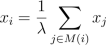, where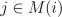means that the sum is over all j such that the nodes i, j are connected. Another way to write this formula is using the adjacency matrix. If we let x be the vector that has the centrality score of node i in component i, then the above equation can be rewritten as the equation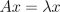. In general, the matrix A will have multiple eigenvalues (the number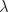is called an eigenvalue), but the Perron-Frobenius theorem guarantees that if we demand all the components of the eigenvector x be positive, then there is only one eigenvalue that satisfies this requirement. Therefore we can assign a unique centrality score to each node.

Activity 3: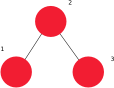1. Figure out the adjacency matrix A for this graph.
2. Use the equationto determine the centrality scores for the nodes of this graph. (Hint: if you have a computer calculation system like Mathematica or a fancy enough calculator, you can use the computer to calculate the eigenvalues and eigenvectors of a matrix and save yourself some time.)
3. Do the centrality scores for the nodes accomplish our goal of giving more connected nodes higher scores?
4. Do the same thing for the graph at the top of the page. For this you'll definitely want to use a computer to calculate the eigenvalues and eigenvectors.